## Abstract

A new damage feature index is presented for the structural health monitoring based on Hilbert-Huang transform (HHT). The energy marginal spectrum of the dynamic signal is used to construct damage characteristic parameter, which can reflect the signal energy variation and benefit the structural damage detection. A sinusoidal wave with frequency change and a composite plate vibration experiment with pre-defined damage are designed to verify the effectiveness of characteristic parameter in damage detection. Results obtained from simulation and test show that the extracted non-model-based damage feature index is available and sensitive in damage detection of time-varying system.

## 1. Introduction

Structural damage induces the change of structural mechanical properties which can affect the stability and lifetime of structures in service. This is why the structural health monitoring (SHM) technology, especially for the critical unit in structure with large scale, has attracted an increasing attention in recent years. In addition, the composites need to pay more attention since the structures are extensively used in engineering field while prone to be damaged .

Damage detection technology is the major part in SHM which can early warn in the initial damage condition to avoid failure of structure, in which the signal processing method is playing an increasingly important role. Among the signal processing methods, the wavelet transform [2-4] and the Hilbert-Huang transform (HHT)[5, 6] are the most typical representatives. W.L. Bayissa  developed a structural damage method based on energy density function using wavelet transform. However, the wavelet transform relies on the choice of wavelet transform which limits the application in engineering.

HHT is composed of empirical mode decomposition (EMD) and Hilbert transform. Compared with the Fourier transform and the wavelet transform, EMD in HHT does not need prior functions . Owing to the adaptive nature, HHT can be used in analyzing nonlinear and non-stationary signals . R. Jha  processed a multi-level structural health monitoring based on HHT and succeeded in detecting and locating the damage. C.X. Bao  improved the HHT algorithm to identify the time-varying systems. The results of simulation and composite beam test showed that the improved algorithm was effective for the analysis of nonlinear signals. M. Bransch  calculated elastic-plastic building ground deformations and failure based on FEM, nonlinear HHT-alpha method and scaled boundary FEM. Pines  used the empirical mode decomposition and the Hilbert phase information to detect structural damage which is proved to be feasible. Through the scholars’ achievements, signal processing methods based on HHT and improved HHT show that HHT has broad prospects whether in system detection  or in structural damage detection [15-18]. However, there still remains great potential of HHT in SHM.

In parallel with the damage detection in SHM, the data acquisition system and experiment system  are also important. N. Roveri  performed the structural health monitoring of railway track and train wheels with the help of a FBG(Fiber Bragg grating) sensor array system. Z. Xia  investigated a multi-agent system based SHM to achieve the compatibility of different kinds of sensors in monitoring of large scale engineering structures. K. Masayuki  evaluated the feasibility of outside strain monitoring method by finite element analyses and experiments. With the advantage of high sensitivity and accuracy, FBG sensor has more and more extensive application in experiment systems and SHM of large-span structures.

HHT is employed here to get the energy marginal spectrum of signal which can reflect the change of structural mechanical properties. A new damage characteristic parameter is constructed based on the energy marginal spectrum. To verify the proposed damage feature index, it is applied in processing a sinusoidal wave signal with frequency change and identifying pre-defined damage in composite plate vibration experiment. Meanwhile, FBG sensors are used in composites experiment to collect the acceleration data. It is proved that the constructed damage feature index is effective and reliable for detecting structural damage of composites.

## 2. The Hilbert-Huang Transform

The HHT method is introduced here in which the concept of instantaneous frequency is applied. According to this theory, any arbitrary time series are composed of intrinsic mode functions (IMFs), which are different with each other, simple and non-sine. Through the empirical mode decomposition (EMD), an intricate time series can be decomposed into several basic time series from high frequency to low frequency, termed IMFs. After the IMFs of Hilbert transforming, the time-frequency spectrum of original signal is got and the analysis in time domain can proceed. To make sure the above process progress smoothly, each IMF have to satisfy the following conditions: first, the number of extremum and zero-crossings of signal can differ by no more than one; second, the mean value of the upper and lower envelopes defined by the local maxima and the local minima should be zero at any point.

The EMD method is the first step of HHT to decompose non-stationary signal. For a given time series ${\textstyle x(t)}$, the process of EMD is introduced as follows. (a)All local extremums are detected firstly, and produce the upper envelope ${\textstyle {x}_{max}}$ and the lower envelope ${\textstyle {x}_{min}}$ by spline approximations using the local maxima and the local minima; (b)obtain ${\textstyle {h}_{1}=}$$x\left(t\right)-({x}_{max}+{x}_{min})/2$, and restart the process by replacing ${\textstyle {h}_{1}}$ with the original signal if ${\textstyle {h}_{1}}$ does not meet the above IMF criteria; (c) if ${\textstyle {h}_{1}}$ is an IMF, regard ${\textstyle {r=x\left(t\right)-h}_{1}}$ as a new ${\textstyle x(t)}$ and repeat the previous steps till ${\textstyle r}$ becomes a monotonic function or cannot decompose into another IMF any more. Based on the decomposition of the original signal so far, ${\textstyle x(t)}$ can be expressed as Eq.(1).

 ${\textstyle x\left(t\right)=\sum _{i=1}^{n}{h}_{i}+r}$
(1)

where ${\textstyle \sum _{i=1}^{n}{h}_{i}}$ is the sum of all IMFs, and ${\textstyle r}$ is the residual component. The IMF expresses a simple oscillation style relative to the harmonic function, which is more general.

After the EMD of ${\textstyle x(t)}$, Hilbert Transform(HT) is the next step. The HT of ${\textstyle x(t)}$ is defined as the convolution of signal and ${\textstyle {\frac {1}{\pi }}}$, which is

 ${\textstyle y\left(t\right)={\frac {1}{\pi }}P\int _{-\infty }^{+\infty }{\frac {x(\tau )}{t-\tau }}{d}_{\tau }}$
(2)

Then the analytical signal is obtained

 ${\textstyle z\left(t\right)=x(t)+iy(t)=A(t){e}^{i\theta (t)}}$
(3)

in which ${\textstyle A(t)}$ is the instantaneous amplitude and ${\textstyle \theta (t)}$ is the phase angle as shown in Eq.(4-5).

 ${\textstyle A\left(t\right)={\sqrt {{x}^{2}\left(t\right)+{y}^{2}(t)}}}$
(4)
 ${\textstyle \theta \left(t\right)=\mathrm {arctan} \,\left({\frac {y(t)}{x(t)}}\right)}$
(5)

The instantaneous frequency is defined as

 ${\textstyle \omega \left(t\right)={\frac {d\theta (t)}{dt}}}$
(6)

Intuitively, the instantaneous frequency is the differential coefficient of the phase of an analytic signal. The signal is decomposed to the components with local symmetric firstly to get the right instantaneous frequency in HHT.

Through applying the Hilbert transform to each IMF component, the Hilbert amplitude spectrum is designated as

 ${\textstyle H\left(\omega ,t\right)=Re\sum _{j=1}^{n}{A}_{j}(t)\mathrm {exp} \,(i\int _{}^{}{\omega }_{j}(t)dt)}$
(7)

which represents the time-frequency distribution of the amplitude of signal. The instantaneous frequency can also be computed according to Eq. (6). Also, the Hilbert marginal spectrum is defined as

 ${\textstyle h\left(\omega \right)=\int _{0}^{T}H(\omega ,t)dt}$
(8)

and the energy marginal spectrum is

 ${\textstyle E\left(\omega \right)=\int _{0}^{T}{H}^{2}(\omega ,t)dt}$
(9)

Compared with the Fourier expansion, the HHT marginal spectrum represents the distribution of signal components in different frequencies. The spectrum value of amplitude and the energy of signal in each frequency band are ${\textstyle h\left({\omega }_{i}\right)}$ and ${\textstyle E\left({\omega }_{i}\right)}$ , ${\textstyle i=}$$1,n$, respectively.

## 3. Damage detection method

Whether the structure is damaged or not can be reflected by the change of response energy in different states. Compared with the amplitude of signal in marginal spectrum, the energy marginal spectrum magnifies the change of response energy generated by structural damage, which is more favorable for the small damage detection.

 ${\textstyle V=\sum _{i=1}^{n}\left|{\frac {{E}^{u}\left({\omega }_{i}\right)-{E}^{d}\left({\omega }_{i}\right)}{\mathrm {max} \,[{E}^{u}\left({\omega }_{i}\right)]}}\right|\times 100\%}$
(10)

In which ${\textstyle {E}^{u}\left({\omega }_{i}\right)}$ and ${\textstyle {E}^{d}\left({\omega }_{i}\right)}$ are the Hilbert energy of dynamic signal before and after the structure are damaged, respectively. Through Eq. (10), we can see that there is no change before and after the signal energy when the value of ${\textstyle V}$ is 0, which means that structural damage is nonexistent. However, the structural damage occurred when the value does not equal to 0. The bigger value means the greater difference between the signal energy of intact and testing structure, and the damage is more likely to happen. So the structural damage can be indicated by the value of signal energy.

## 4. Numerical simulation

In this simulation, a 10s sinusoidal wave with a sudden frequency change at 5s from 1Hz to 1.1Hz is analyzed. Through EMD, the original signal is resolved into 4 IMFs and residue. Original signal and IMFs are shown in Fig. 1. The instantaneous frequency of IMFs and Hilbert spectrum are obtained and given in Figs. 2-3 through HT. As shown in Fig. 2, the frequency shift at 5s can be directly captured from instantaneous frequency of the first IMF while the shifts of other IMFs are not obvious. The change of amplitude in Hilbert spectrum also reflects the frequency shift of signal at 5s.

After the Hilbert energy is obtained, the value of signal energy can be calculated through Eq.10 by comparing two signals in different conditions. In the first case, the original signal is divided into two parts, which is the signal of front 5s and last 5s, respectively. The value of ${\textstyle V}$ is 9.23%, which means that the signal parameter of last 5s has changed compared with the signal of front 5s.In the second case, the 10s signal of 1Hz is compared with the original signal. In this condition the value of ${\textstyle V}$ is 3.7%, which also means that the character of two signals is different and structural damage occurs.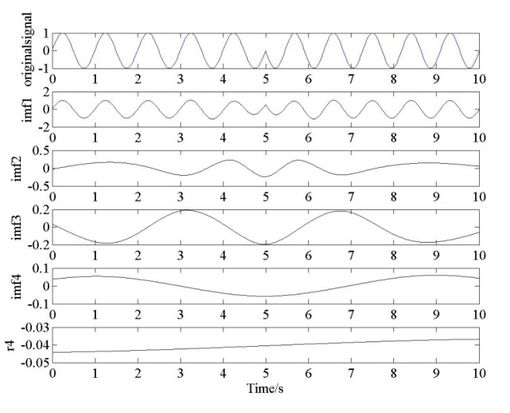Figure 1. Original sine wave and IMFs.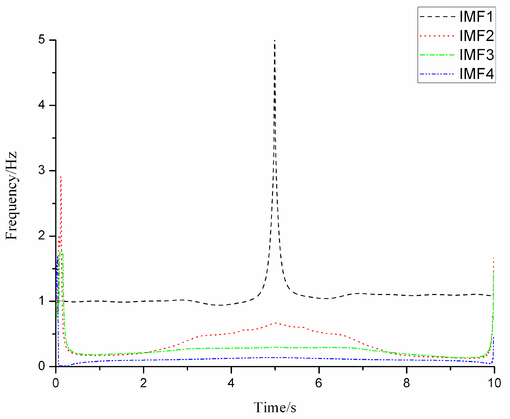Figure 2. Instantaneous frequency of the IMFs.

## 5. Experimental verification

In Section 4, the parameter based on HHT was used to identify the change of a sinusoidal wave which was proved to be effective. To test the structural damage detection method, a vibration experiment of composite plate form wing skin was designed and performed.

Composite material used in test was T300/QY8911 and the material property is shown in Table 1. The thickness of single layer was 0.18mm and stacking sequence of composite laminates was ${\textstyle {[45/-45/0/-45/45/0]}_{S}}$. One side of the ${\textstyle 200\times 2000\times 2.16}$ mm3 composite plate was clamped to vibrating table. There were two symmetrical isosceles triangular breaches near the clamped end. The acceleration of vibrating table was 4g and the frequency of vibration was 74Hz.

Table 1. Material properties of T300/QY8911 (MPa)
 ${E}_{XX}$ ${E}_{YY}$ ${E}_{ZZ}$ ${G}_{XY}$ ${G}_{XZ}$ ${G}_{YZ}$ ${v}_{XY}$ ${v}_{XZ}$ ${v}_{YZ}$ 135000 8800 8800 4470 4470 3200 0.33 0.33 0.48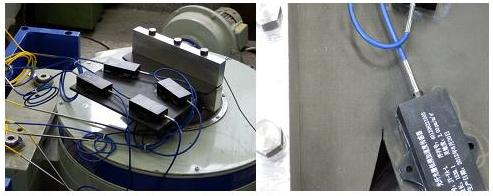Figure 4. Composite plate with pre-defined damage.

As shown in Fig. 4, a crack in one breach was made to be pre-defined damage. Structural damage was detected and located by comparing the signal of damaged structure with the intact one. The sample frequency was 1000Hz. Acceleration sensors were distributed as shown in Fig. 5. The wavelengths of FBG sensors are 1541nm, 1555nm, 1538nm and 1558nm, respectively.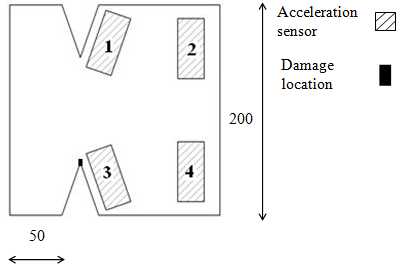Figure 5. Sensor set and damage location.

Taking the acceleration data of sensor 4 for example, HHT is employed in signal processing of intact structure and damaged one. The results of 0.5s signal in two cases are plotted in Figs. 6-9. As shown in Fig. 6, two original signals were decomposed into 4 IMFs respectively. From the instantaneous frequency given in Fig. 7, it can be found that there is a little end-effect around boundary area in IMF of low frequency which is ignorable since the energy of these frequencies is quite low. It is hard to find the difference between the two HHT spectrums from Fig. 8. The marginal energy of intact laminate ranges from 40Hz to 100Hz, while the damaged one ranges from 50Hz to 100Hz are shown in Fig. 9.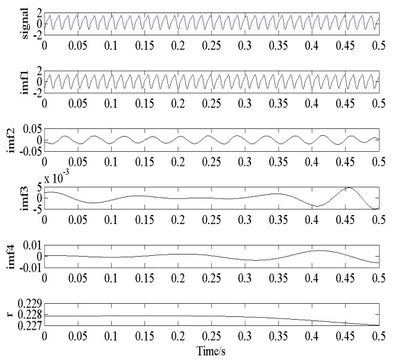(a) IMFs of intact laminate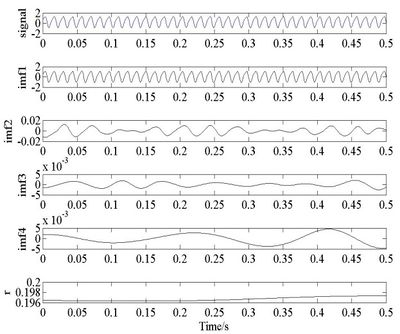(b) IMFs of damaged laminate Figure 6. EMD results of structural signals.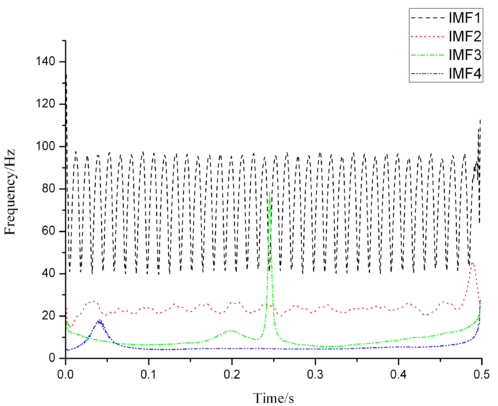(a) Instantaneous frequency of intact laminate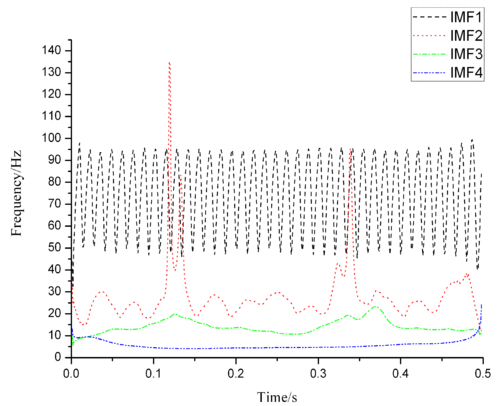(b) Instantaneous frequency of damaged laminate Figure 7. Instantaneous frequency of structural signals.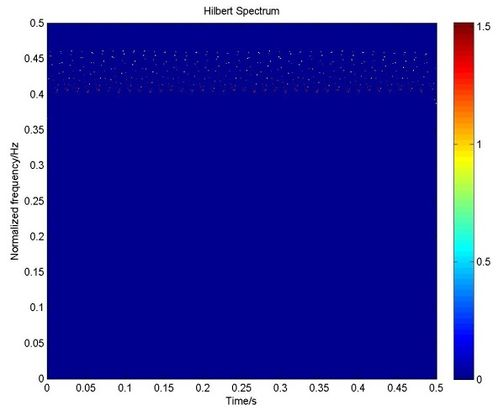(a) HHT spectrum of intact laminate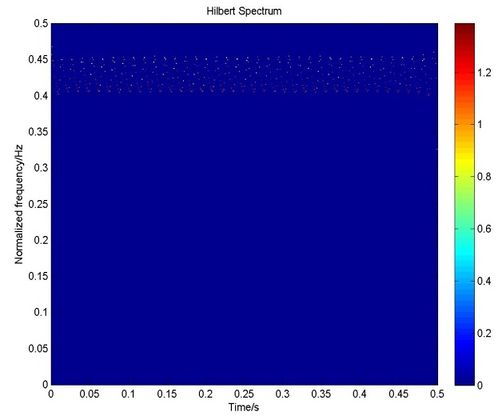(b) HHT spectrum of damaged laminate Figure 8. HHT spectrum of structural signals.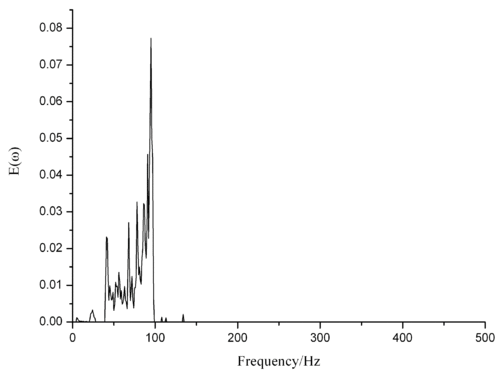(a) Energy marginal spectrum of intact laminate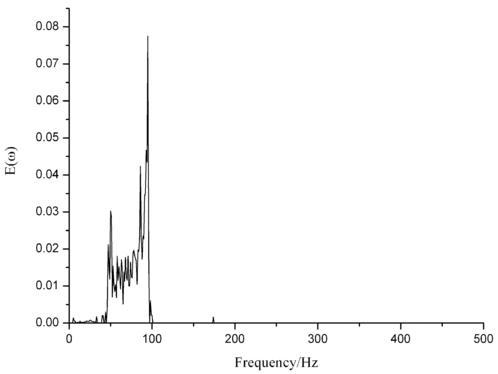(b) Energy marginal spectrum of damaged laminate Figure 9. Energy marginal spectrum of structural signals.

Based on Eq. (10), the change of signal energy can be obtained as 6.63%. Since ${\textstyle V}$ of sensor 4 signal is greater than 0, structural damage has occurred. However, the location of damage has not been captured yet. ${\textstyle V}$ of structural signals are shown in Table 2. From comparing the change of signal energy, ${\textstyle V}$ of sensor 3 signal is bigger which means that the structural damage is more likely to happen around this sensor. The damage detection results are in consistent with the pre-defined damage condition.

Table 2. V of structural response signals
 Sensor 1 Sensor 2 Sensor 3 Sensor 4 V 3.02% 1.47% 13.7% 6.63%

## 6. Conclusions

The Hilbert-Huang transformation is an effective method for analyzing nonlinear and non-stationary signal. A new structural damage detection parameter based on the Hilbert-Huang transformation is developed here. The damage characteristic value obtained from Hilbert energy spectrum is used to identify structural damage in a sinusoidal wave with a sudden frequency change and a composite plate with pre-defined damage. The change of signal energy is got through comparing the signal energy of intact and damaged structural which can implement the detection and localization of structural damage. Through the results of simulation and experiment, the following conclusions can be drawn: 1) the Hilbert spectrum energy can reflect the distribution of signal energy and the constructed structural damage feature index can be used in identifying structural damage and localizing the damage; 2) signal energy variation is sensitive to identify small damage without specific structural parameter which satisfies the requirement of structural health monitoring of dynamic and real-time system; 3) the size of FBG acceleration sensor used in composites vibration test is quite relative to test sample, which can be substituted by sensor with smaller volume in future research such as strain sensor. After the detection and localization of structural damage, the assessment of damage level is the future goal. Meanwhile, this parameter needs to be further improved which includes eliminating the end-effect in EMD.

## Acknowledgements

This study is supported by the National Natural Science Foundation (No: 51475369).

### Document informationPublished on 03/01/18
Accepted on 26/10/17
Submitted on 12/07/17

Volume 34, Issue 1, 2018
DOI: 10.23967/j.rimni.2017.9.002

### Document Score0

Views 264
Recommendations 0